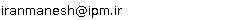••••••••## “Ali Iranmanesh”Email:### IPM Positions

Non Resident Researcher (non-resident), School of Mathematics
(2005 - 2006 )

### Past IPM Positions

Associate Researcher (non-resident), School of Mathematics
(2000 - 2005)

### Non IPM Affiliations

Associate Professor of Tarbiat Modares University

### Research Activities

If n is an integer, then p(n) is the set of prime divisors of n and if G is a finite group p(G) is defined to be p(|G|). The prime graph G(G) of a group G is a graph whose vertex set is p(G), and two distinct primes p and q are linked by an edge if and only if G contains an element of order pq. Let pi, i=1,2,..., t(G) be the connected components of G(G). For |G| even, p1 will be the connected component containing 2. Then |G| can be expressed as a product of some positive integers mi, i=1,2,...,t(G) with p(mi)=pi. The integers mi's are called the order components of G. The set of order components of G will be denoted by OC(G). If the order of G is even, we will assume that m1 is the even order component and m2,...,mt(G) will be the odd order components of G. The order components of non-abelian simple groups having at least three prime graph components are obtained by G. Y. Chen. The following groups are uniquely determined by their order components : G2(q) where q ? 0 (mod 3), Sporadic simple groups, Suzuki-Ree groups, E8(q), PSL(n,q) for n=2,3,5, Ap where p and p-2 are primes, and F4(q).

In this research program , we prove that groups PSU(n,q) for some n and q are also uniquely determined by their order components.

AMS Subject Classification: 20D05,20D60

Keywords : Prime graph, order component, finite group, simple group .

### Present Research Project at IPM

A Characterization of PSU(n,q) for some n and q

### Related Papers

 1. Nonrigid group theory of ammonia tetramer: (NH3)4Match 56 (2006), 317-330  [abstract]
 2. A study of the restricted non-rigid group of tetra metyl tangstan hydridAsian. J. Chem. (Accepted) [abstract]
 3. Full-non-rigid group theory for Hepta methyl tungstenInt. J. Pure Appl. Math. (Accepted) [abstract]
 4. A characterization of PSU(23, q)Int. J. Appl. Math. 22 (2005), 449-461  [abstract]
 5. M. Dabirian and A. Iranmanesh The full non-rigid group theory for Trimethylamine-BH3 AddendMatch 54 (2005), 75-88  [abstract]
 6. A characterization of PSU(17, q)J. Appl. Algebra Discrete Struct. 3 (2005), 169-188  [abstract]
 7. A characterization of C4(q) where q = 2nChinese J. Contemp. Math. 26 (2005), 105-110  [abstract]
 8. A characterization of PSU11(q)Canad. Math. Bull. 47 (2004), 530-539  [abstract]
 9. A characterization of PSU(19,q)Int. J. Pure Appl. Math. 15 (2004), 499-511  [abstract]
 10. A characterization of PSU5(q)Int. Math. J. 3 (2003), 129-141  [abstract]
 11. A characterization of PSU3(q) for q > 5Southeast Asian Bull. Math. 26 (2002), 33-44  [abstract]
 12. A characterization of C2(q) where q > 5Comment. Math. Univ. Carolin. 43 (2002), 9-21  [abstract]
 13. A. Iranmanesh and S.H. Alavi A characterisation of simple groups PSL(5,q)Bull. Aust. Math. Soc. 65 (2002), 211-222  [abstract]
 14. A. Iranmanesh, S.H. Alavi and B. Khosravi A characterization of PSL (3,q) for q=2mActa Math. Sci. Ser. B Engl. Ed. 18 (2002), 463-472  [abstract]
 15. B. Khosravi, A. Iranmanesh and S.H. Alavi A characterization of PSL(3,q) where q is an odd prime powerJ. Pure Appl. Algebra 170 (2002), 243-254  [abstract]
 16. A. Iranmanesh and S. H. Alavi A new characterization of Ap where p and p−2 are primesKorean J. Comput. Appl. Math. 8 (2001), 665-673  [abstract]
 17. A. Iranmanesh and B. Khosravi A characterization of F4(q) where q=2n(n > 1)Far East J. Math. Sci. 2 (2000), 853-859  [abstract]
 18. General types of conjugacy classes of GLn(q)Far East J. Math. Sci. 2 (2000), 93-103  [abstract]
 [Back]
scroll left or right